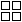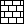# Common Tiles node

Left Toolbar >Layout Nodes >Common Tiles

The Common Tiles node creates a seamless tile pattern following a common bricks pattern. This node takes as input an image with the tile sources and layers them in rows or columns with offset and slight random variations.

inputinput image

outputoutput image

## Procedure

### To create a seamless common tile pattern:

1. On the left toolbar choose Layout Nodes > Common Tiles.>2. Drag the Common Tiles icon into the Node Graph.3. Connect the output of the images to be laid out as tiles by the common tiles node.

## Attributes Panel### Input

*Num Tiles *
Number of tiles in x and y in the input images.

Symmetry Symmetry of the input tiles.
None: The input tiles cannot be rotated.
Rectangle: The input tiles can be rotated in 180° steps.

Triangle: The input tiles can be rotated in 180° steps.

Square: The input tiles can be rotated in 90° steps.

Hexagons: The input tiles can be rotated in 180° steps.

### Output

Position
Position X and Y of the output image.

Num Tiles
Number of tiles in X and Y of the output image.

Constrain

Criteria to constrain the output tiles.

Output Size

Tile Size

Distance

*Output Size *
Size X and Y of the output image.

Gap

Gap in X and Y between output tiles.

Offset

Offset direction in X and Y of the output tiles.

### Random

Seed
Seed for all the random variations.

Rotation
Adds random variations to the rotation of the output tiles. This values is relative to the Symmetry of the input tiles. A square tile can have a random rotation of maximum 90°. A rectangular tile can have a random rotation of maximum 180°.

Scale
Adds random variations to the scale of the output tiles.

Translation
Adds random variations to the translation in X and Y of the output tiles.

Hue
Adds random variations to the hue of the color of the output tiles.
This attribute has effect only on RGB and RGBA images.

Saturation
Adds random variations to the saturation of the color of the output tiles.
This attribute has effect only on RGB and RGBA images.

Value
Adds random variations to the value of the color of the output tiles.
This attribute has effect only on RGB and RGBA images.

You might also be interested in: